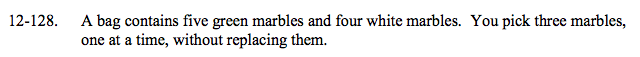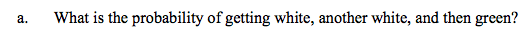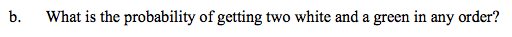### Home > A2C > Chapter 12 > Lesson 12.3.1 > Problem12-128

12-128.$\frac{4}{9}\cdot(?)(?)$

Remember that the marbles are not replaced, so the probabilities for drawing the next marbles are changed.Use combinations.

How many ways can you draw two white marbles and one green marble? How many ways can you draw three marbles?